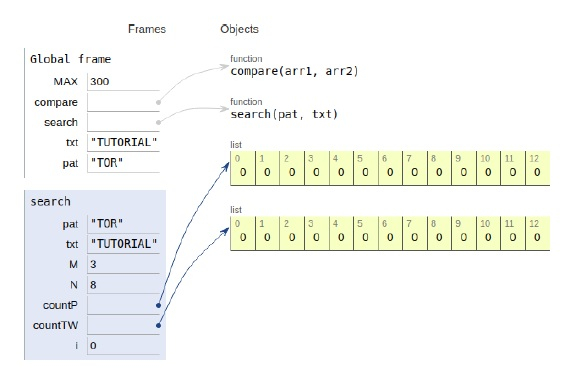# Python Program for Anagram Substring Search

Problem statement − Given a text and a pattern, we need to print all occurrences of pattern and its permutations (or anagrams) in text.

Now let’s observe the solution in the implementation below −

## Example

Live Demo

# maximum value
MAX = 300
# compare
def compare(arr1, arr2):
for i in range(MAX):
if arr1[i] != arr2[i]:
return False
return True
# search
def search(pat, txt):
M = len(pat)
N = len(txt)
# countP pattern account
# countTW text window count
countP = *MAX
countTW = *MAX
for i in range(M):
(countP[ord(pat[i]) ]) += 1
(countTW[ord(txt[i]) ]) += 1
# Traversal
for i in range(M, N):
# Compare current window and patten counts
if compare(countP, countTW):
print("Found at Index", (i-M))
(countTW[ ord(txt[i]) ]) += 1
# remove charcter from window
(countTW[ ord(txt[i-M]) ]) -= 1
# Check for the last window
if compare(countP, countTW):
print("It is Found at Index : ", N-M)
# main
txt = "TUTORIALSPOINT"
pat = "TOR"
search(pat, txt)

## Output

Found at Index 2All the variables are declared in the local scope and their references are seen in the figure above.

## Conclusion

In this article, we have learned about how we can make a Python Program for Anagram Substring Search.